Publicité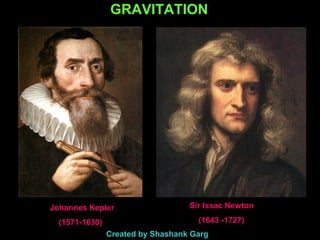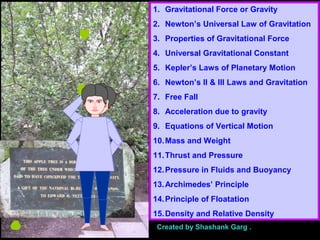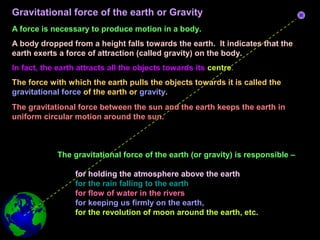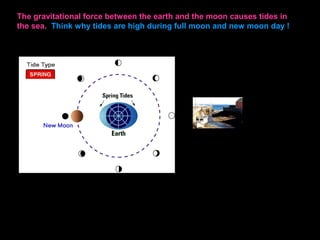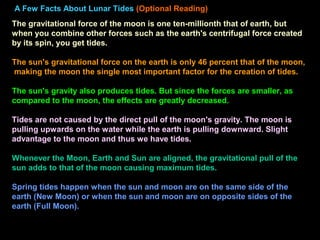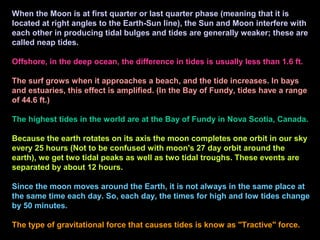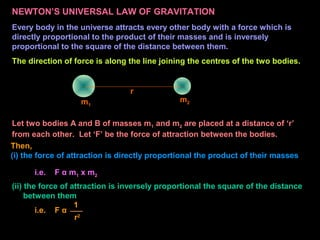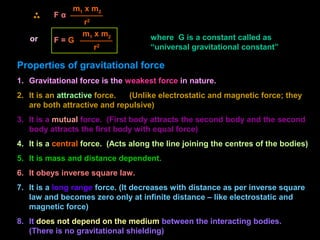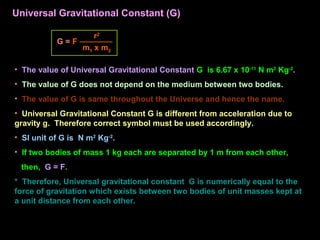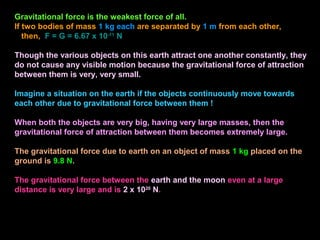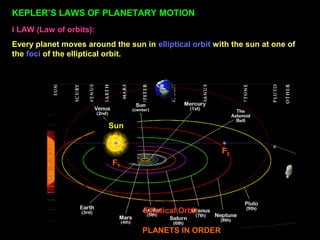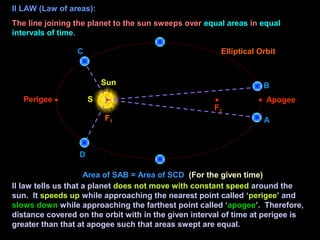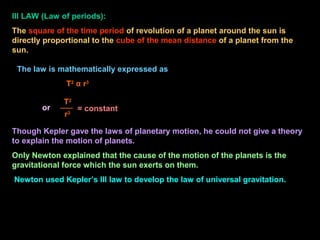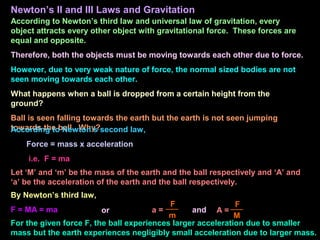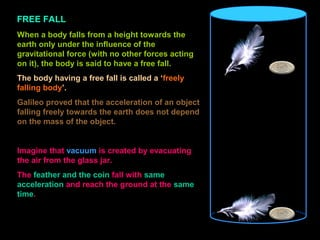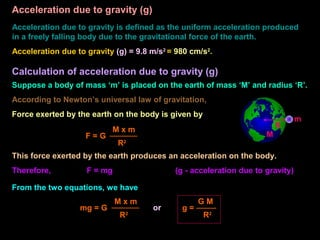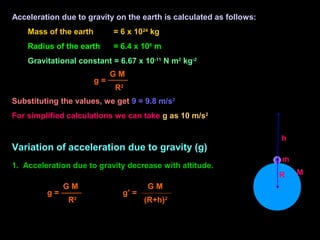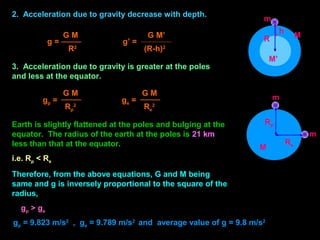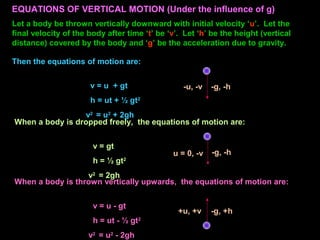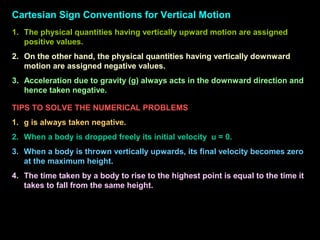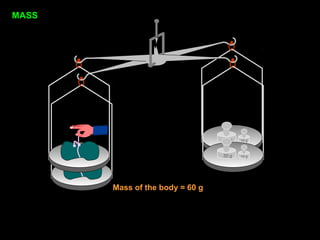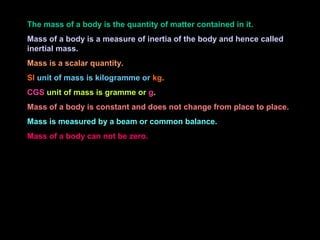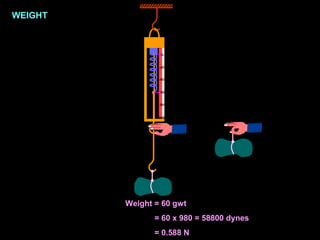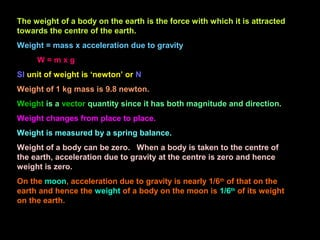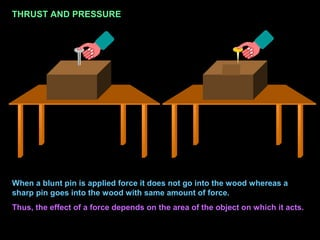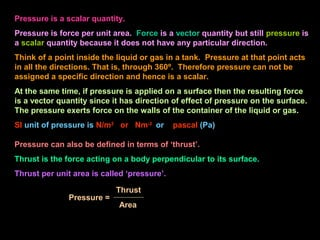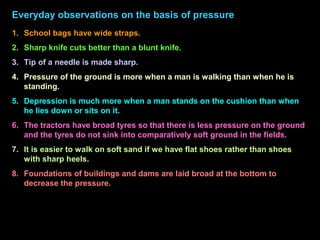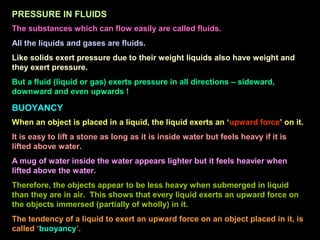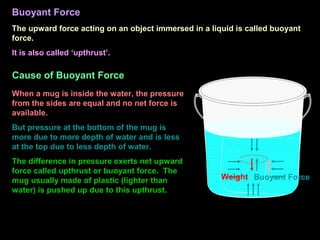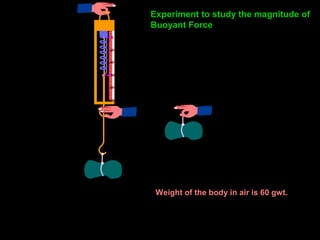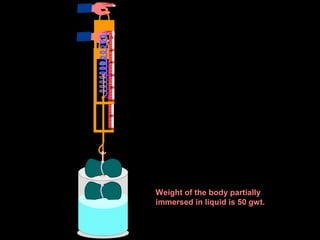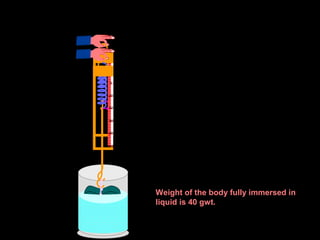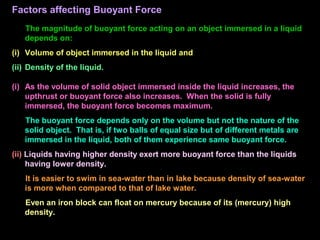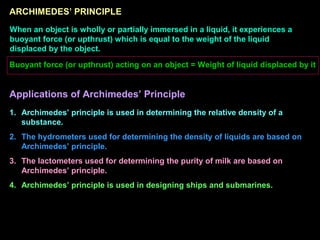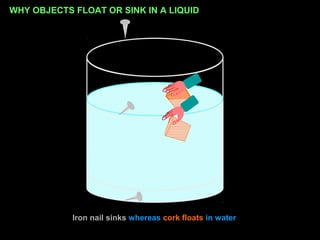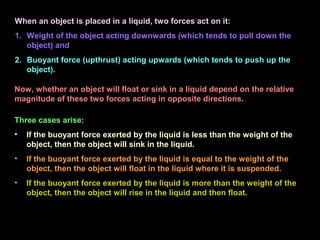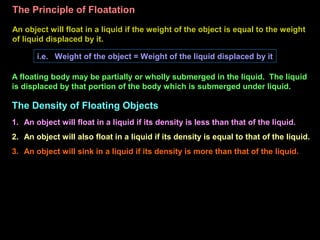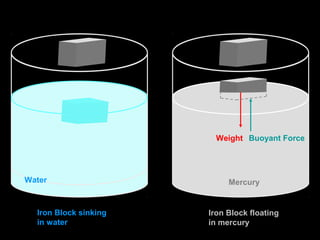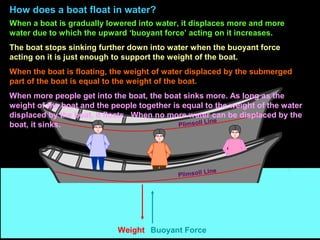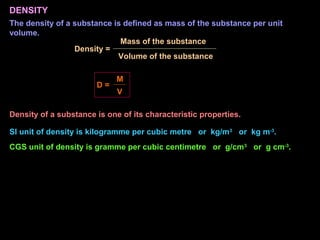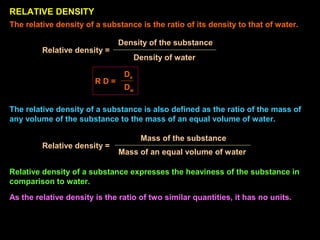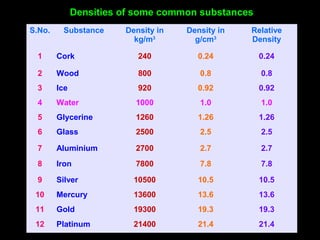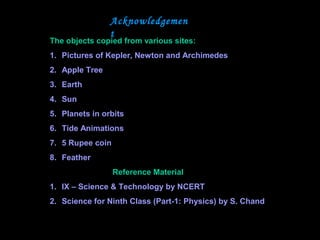1 sur 45
Publicité

### 10 gravitation.pdf

1. GRAVITATION Sir Issac Newton (1643 -1727) Johannes Kepler (1571-1630) Created by Shashank Garg ch 10
2. 1. Gravitational Force or Gravity 2. Newton’s Universal Law of Gravitation 3. Properties of Gravitational Force 4. Universal Gravitational Constant 5. Kepler’s Laws of Planetary Motion 6. Newton’s II & III Laws and Gravitation 7. Free Fall 8. Acceleration due to gravity 9. Equations of Vertical Motion 10.Mass and Weight 11.Thrust and Pressure 12.Pressure in Fluids and Buoyancy 13.Archimedes’ Principle 14.Principle of Floatation 15.Density and Relative Density Created by Shashank Garg .
3. Gravitational force of the earth or Gravity A force is necessary to produce motion in a body. A body dropped from a height falls towards the earth. It indicates that the earth exerts a force of attraction (called gravity) on the body. In fact, the earth attracts all the objects towards its centre. The force with which the earth pulls the objects towards it is called the gravitational force of the earth or gravity. The gravitational force between the sun and the earth keeps the earth in uniform circular motion around the sun. The gravitational force of the earth (or gravity) is responsible – for holding the atmosphere above the earth for the rain falling to the earth for flow of water in the rivers for keeping us firmly on the earth, for the revolution of moon around the earth, etc.
4. The gravitational force between the earth and the moon causes tides in the sea. Think why tides are high during full moon and new moon day !
5. A Few Facts About Lunar Tides (Optional Reading) The gravitational force of the moon is one ten-millionth that of earth, but when you combine other forces such as the earth's centrifugal force created by its spin, you get tides. The sun's gravitational force on the earth is only 46 percent that of the moon, making the moon the single most important factor for the creation of tides. The sun's gravity also produces tides. But since the forces are smaller, as compared to the moon, the effects are greatly decreased. Tides are not caused by the direct pull of the moon's gravity. The moon is pulling upwards on the water while the earth is pulling downward. Slight advantage to the moon and thus we have tides. Whenever the Moon, Earth and Sun are aligned, the gravitational pull of the sun adds to that of the moon causing maximum tides. Spring tides happen when the sun and moon are on the same side of the earth (New Moon) or when the sun and moon are on opposite sides of the earth (Full Moon).
6. When the Moon is at first quarter or last quarter phase (meaning that it is located at right angles to the Earth-Sun line), the Sun and Moon interfere with each other in producing tidal bulges and tides are generally weaker; these are called neap tides. Offshore, in the deep ocean, the difference in tides is usually less than 1.6 ft. The surf grows when it approaches a beach, and the tide increases. In bays and estuaries, this effect is amplified. (In the Bay of Fundy, tides have a range of 44.6 ft.) The highest tides in the world are at the Bay of Fundy in Nova Scotia, Canada. Because the earth rotates on its axis the moon completes one orbit in our sky every 25 hours (Not to be confused with moon's 27 day orbit around the earth), we get two tidal peaks as well as two tidal troughs. These events are separated by about 12 hours. Since the moon moves around the Earth, it is not always in the same place at the same time each day. So, each day, the times for high and low tides change by 50 minutes. The type of gravitational force that causes tides is know as "Tractive" force.
7. NEWTON’S UNIVERSAL LAW OF GRAVITATION Every body in the universe attracts every other body with a force which is directly proportional to the product of their masses and is inversely proportional to the square of the distance between them. The direction of force is along the line joining the centres of the two bodies. r m1 m2 Let two bodies A and B of masses m1 and m2 are placed at a distance of ‘r’ from each other. Let ‘F’ be the force of attraction between the bodies. i.e. F α m1 x m2 (ii) the force of attraction is inversely proportional the square of the distance between them i.e. F α 1 r2 Then, (i) the force of attraction is directly proportional the product of their masses
8. or F α m1 x m2 r2 where G is a constant called as “universal gravitational constant” F = G m1 x m2 r2 Properties of gravitational force 1. Gravitational force is the weakest force in nature. 2. It is an attractive force. (Unlike electrostatic and magnetic force; they are both attractive and repulsive) 3. It is a mutual force. (First body attracts the second body and the second body attracts the first body with equal force) 4. It is a central force. (Acts along the line joining the centres of the bodies) 5. It is mass and distance dependent. 6. It obeys inverse square law. 7. It is a long range force. (It decreases with distance as per inverse square law and becomes zero only at infinite distance – like electrostatic and magnetic force) 8. It does not depend on the medium between the interacting bodies. (There is no gravitational shielding)
9. • The value of Universal Gravitational Constant G is 6.67 x 10-11 N m2 Kg-2 . • The value of G does not depend on the medium between two bodies. • The value of G is same throughout the Universe and hence the name. • Universal Gravitational Constant G is different from acceleration due to gravity g. Therefore correct symbol must be used accordingly. • SI unit of G is N m2 Kg-2 . • If two bodies of mass 1 kg each are separated by 1 m from each other, then, G = F. * Therefore, Universal gravitational constant G is numerically equal to the force of gravitation which exists between two bodies of unit masses kept at a unit distance from each other. G = F m1 x m2 r2 Universal Gravitational Constant (G)
10. Gravitational force is the weakest force of all. If two bodies of mass 1 kg each are separated by 1 m from each other, then, F = G = 6.67 x 10-11 N Though the various objects on this earth attract one another constantly, they do not cause any visible motion because the gravitational force of attraction between them is very, very small. Imagine a situation on the earth if the objects continuously move towards each other due to gravitational force between them ! When both the objects are very big, having very large masses, then the gravitational force of attraction between them becomes extremely large. The gravitational force due to earth on an object of mass 1 kg placed on the ground is 9.8 N. The gravitational force between the earth and the moon even at a large distance is very large and is 2 x 1020 N.
11. KEPLER’S LAWS OF PLANETARY MOTION I LAW (Law of orbits): Every planet moves around the sun in elliptical orbit with the sun at one of the foci of the elliptical orbit. PLANETS IN ORDER F1 F2 Sun Elliptical Orbit
12. F1 F2 Sun S F1 F2 Sun S D C A B Elliptical Orbit II LAW (Law of areas): The line joining the planet to the sun sweeps over equal areas in equal intervals of time. Apogee Perigee II law tells us that a planet does not move with constant speed around the sun. It speeds up while approaching the nearest point called ‘perigee’ and slows down while approaching the farthest point called ‘apogee’. Therefore, distance covered on the orbit with in the given interval of time at perigee is greater than that at apogee such that areas swept are equal. Area of SAB = Area of SCD (For the given time)
13. III LAW (Law of periods): The square of the time period of revolution of a planet around the sun is directly proportional to the cube of the mean distance of a planet from the sun. Though Kepler gave the laws of planetary motion, he could not give a theory to explain the motion of planets. Only Newton explained that the cause of the motion of the planets is the gravitational force which the sun exerts on them. Newton used Kepler’s III law to develop the law of universal gravitation. = constant T2 r3 The law is mathematically expressed as T2 α r3 or
14. Newton’s II and III Laws and Gravitation According to Newton’s third law and universal law of gravitation, every object attracts every other object with gravitational force. These forces are equal and opposite. Therefore, both the objects must be moving towards each other due to force. However, due to very weak nature of force, the normal sized bodies are not seen moving towards each other. What happens when a ball is dropped from a certain height from the ground? Ball is seen falling towards the earth but the earth is not seen jumping towards the ball. Why? According to Newton’s second law, Force = mass x acceleration i.e. F = ma Let ‘M’ and ‘m’ be the mass of the earth and the ball respectively and ‘A’ and ‘a’ be the acceleration of the earth and the ball respectively. By Newton’s third law, F = MA = ma a = F m or A = F M and For the given force F, the ball experiences larger acceleration due to smaller mass but the earth experiences negligibly small acceleration due to larger mass.
15. FREE FALL When a body falls from a height towards the earth only under the influence of the gravitational force (with no other forces acting on it), the body is said to have a free fall. The body having a free fall is called a ‘freely falling body’. Galileo proved that the acceleration of an object falling freely towards the earth does not depend on the mass of the object. Imagine that vacuum is created by evacuating the air from the glass jar. The feather and the coin fall with same acceleration and reach the ground at the same time.
16. Acceleration due to gravity (g) Acceleration due to gravity is defined as the uniform acceleration produced in a freely falling body due to the gravitational force of the earth. Acceleration due to gravity (g) = 9.8 m/s2 = 980 cm/s2 . Calculation of acceleration due to gravity (g) Suppose a body of mass ‘m’ is placed on the earth of mass ‘M’ and radius ‘R’. According to Newton’s universal law of gravitation, Force exerted by the earth on the body is given by R m M F = G M x m R2 This force exerted by the earth produces an acceleration on the body. Therefore, F = mg (g - acceleration due to gravity) From the two equations, we have mg = G M x m R2 or g = G M R2
17. Acceleration due to gravity on the earth is calculated as follows: Mass of the earth = 6 x 1024 kg Radius of the earth = 6.4 x 106 m Gravitational constant = 6.67 x 10-11 N m2 kg-2 g = G M R2 Substituting the values, we get 9 = 9.8 m/s2 For simplified calculations we can take g as 10 m/s2 Variation of acceleration due to gravity (g) 1. Acceleration due to gravity decrease with altitude. h m M R g = G M R2 g’ = G M (R+h)2
18. 2. Acceleration due to gravity decrease with depth. g = G M R2 g’ = G M’ (R-h)2 h m 3. Acceleration due to gravity is greater at the poles and less at the equator. gp = G M Rp 2 ge = G M Re 2 m M Rp m Re Earth is slightly flattened at the poles and bulging at the equator. The radius of the earth at the poles is 21 km less than that at the equator. i.e. Rp < Re Therefore, from the above equations, G and M being same and g is inversely proportional to the square of the radius, gp > ge gp = 9.823 m/s2 , ge = 9.789 m/s2 and average value of g = 9.8 m/s2 M M’ R
19. EQUATIONS OF VERTICAL MOTION (Under the influence of g) Let a body be thrown vertically downward with initial velocity ‘u’. Let the final velocity of the body after time ‘t’ be ‘v’. Let ‘h’ be the height (vertical distance) covered by the body and ‘g’ be the acceleration due to gravity. Then the equations of motion are: v = u + gt h = ut + ½ gt2 v2 = u2 + 2gh When a body is dropped freely, the equations of motion are: v = gt h = ½ gt2 v2 = 2gh When a body is thrown vertically upwards, the equations of motion are: v = u - gt h = ut - ½ gt2 v2 = u2 - 2gh -u, -v -g, -h u = 0, -v -g, -h +u, +v -g, +h
20. Cartesian Sign Conventions for Vertical Motion 1. The physical quantities having vertically upward motion are assigned positive values. 2. On the other hand, the physical quantities having vertically downward motion are assigned negative values. 3. Acceleration due to gravity (g) always acts in the downward direction and hence taken negative. TIPS TO SOLVE THE NUMERICAL PROBLEMS 1. g is always taken negative. 2. When a body is dropped freely its initial velocity u = 0. 3. When a body is thrown vertically upwards, its final velocity becomes zero at the maximum height. 4. The time taken by a body to rise to the highest point is equal to the time it takes to fall from the same height.
21. 50 g 10 g 50 g 10 g Mass of the body = 60 g MASS
22. The mass of a body is the quantity of matter contained in it. Mass of a body is a measure of inertia of the body and hence called inertial mass. Mass is a scalar quantity. SI unit of mass is kilogramme or kg. CGS unit of mass is gramme or g. Mass of a body is constant and does not change from place to place. Mass is measured by a beam or common balance. Mass of a body can not be zero.
23. Weight = 60 gwt = 60 x 980 = 58800 dynes = 0.588 N 0 20 40 80 60 100 0 20 40 80 60 100 WEIGHT
24. The weight of a body on the earth is the force with which it is attracted towards the centre of the earth. Weight = mass x acceleration due to gravity W = m x g SI unit of weight is ‘newton’ or N Weight of 1 kg mass is 9.8 newton. Weight is a vector quantity since it has both magnitude and direction. Weight changes from place to place. Weight is measured by a spring balance. Weight of a body can be zero. When a body is taken to the centre of the earth, acceleration due to gravity at the centre is zero and hence weight is zero. On the moon, acceleration due to gravity is nearly 1/6th of that on the earth and hence the weight of a body on the moon is 1/6th of its weight on the earth.
25. Archimedes ( 287 BC – 212 Blaise Pascal (1623 - 1662)
26. THRUST AND PRESSURE When a blunt pin is applied force it does not go into the wood whereas a sharp pin goes into the wood with same amount of force. Thus, the effect of a force depends on the area of the object on which it acts.
27. Weight of a body is also a force and it always acts in the downward direction. Observe the two different positions of brick on the ground. REX REX Both the bricks exert same amount of force on the ground because they have same weight but the two bricks exert different pressure due to different area of contact with the ground. A lying brick exerts less pressure on the ground whereas the standing brick exerts more pressure on the ground. Therefore, pressure depends on (i) Force applied and (ii) Area over which it acts. Pressure is the force acting perpendicularly on a unit area of the object. Pressure = Area Force or P = A F
28. Pressure is a scalar quantity. Pressure is force per unit area. Force is a vector quantity but still pressure is a scalar quantity because it does not have any particular direction. Think of a point inside the liquid or gas in a tank. Pressure at that point acts in all the directions. That is, through 360º. Therefore pressure can not be assigned a specific direction and hence is a scalar. At the same time, if pressure is applied on a surface then the resulting force is a vector quantity since it has direction of effect of pressure on the surface. The pressure exerts force on the walls of the container of the liquid or gas. SI unit of pressure is N/m2 or Nm-2 or pascal (Pa) Pressure can also be defined in terms of ‘thrust’. Thrust is the force acting on a body perpendicular to its surface. Thrust per unit area is called ‘pressure’. Pressure = Area Thrust
29. Everyday observations on the basis of pressure 1. School bags have wide straps. 2. Sharp knife cuts better than a blunt knife. 3. Tip of a needle is made sharp. 4. Pressure of the ground is more when a man is walking than when he is standing. 5. Depression is much more when a man stands on the cushion than when he lies down or sits on it. 6. The tractors have broad tyres so that there is less pressure on the ground and the tyres do not sink into comparatively soft ground in the fields. 7. It is easier to walk on soft sand if we have flat shoes rather than shoes with sharp heels. 8. Foundations of buildings and dams are laid broad at the bottom to decrease the pressure.
30. PRESSURE IN FLUIDS The substances which can flow easily are called fluids. All the liquids and gases are fluids. Like solids exert pressure due to their weight liquids also have weight and they exert pressure. But a fluid (liquid or gas) exerts pressure in all directions – sideward, downward and even upwards ! BUOYANCY When an object is placed in a liquid, the liquid exerts an ‘upward force’ on it. It is easy to lift a stone as long as it is inside water but feels heavy if it is lifted above water. A mug of water inside the water appears lighter but it feels heavier when lifted above the water. Therefore, the objects appear to be less heavy when submerged in liquid than they are in air. This shows that every liquid exerts an upward force on the objects immersed (partially of wholly) in it. The tendency of a liquid to exert an upward force on an object placed in it, is called ‘buoyancy’.
31. Buoyant Force The upward force acting on an object immersed in a liquid is called buoyant force. It is also called ‘upthrust’. Cause of Buoyant Force When a mug is inside the water, the pressure from the sides are equal and no net force is available. But pressure at the bottom of the mug is more due to more depth of water and is less at the top due to less depth of water. The difference in pressure exerts net upward force called upthrust or buoyant force. The mug usually made of plastic (lighter than water) is pushed up due to this upthrust. Buoyant Force Weight
32. 0 20 40 80 60 100 0 20 40 80 60 100 Experiment to study the magnitude of Buoyant Force Weight of the body in air is 60 gwt.
33. 0 20 40 80 60 100 0 20 40 80 60 100 0 20 40 80 60 100 0 20 40 80 60 100 Weight of the body partially immersed in liquid is 50 gwt.
34. 0 20 40 80 60 100 0 20 40 80 60 100 0 20 40 80 60 100 0 20 40 80 60 100 Weight of the body fully immersed in liquid is 40 gwt.
35. Factors affecting Buoyant Force The magnitude of buoyant force acting on an object immersed in a liquid depends on: (i) Volume of object immersed in the liquid and (ii) Density of the liquid. (i) As the volume of solid object immersed inside the liquid increases, the upthrust or buoyant force also increases. When the solid is fully immersed, the buoyant force becomes maximum. The buoyant force depends only on the volume but not the nature of the solid object. That is, if two balls of equal size but of different metals are immersed in the liquid, both of them experience same buoyant force. (ii) Liquids having higher density exert more buoyant force than the liquids having lower density. It is easier to swim in sea-water than in lake because density of sea-water is more when compared to that of lake water. Even an iron block can float on mercury because of its (mercury) high density.
36. ARCHIMEDES’ PRINCIPLE When an object is wholly or partially immersed in a liquid, it experiences a buoyant force (or upthrust) which is equal to the weight of the liquid displaced by the object. Buoyant force (or upthrust) acting on an object = Weight of liquid displaced by it Applications of Archimedes’ Principle 1. Archimedes’ principle is used in determining the relative density of a substance. 2. The hydrometers used for determining the density of liquids are based on Archimedes’ principle. 3. The lactometers used for determining the purity of milk are based on Archimedes’ principle. 4. Archimedes’ principle is used in designing ships and submarines.
37. WHY OBJECTS FLOAT OR SINK IN A LIQUID Iron nail sinks whereas cork floats in water
38. When an object is placed in a liquid, two forces act on it: 1. Weight of the object acting downwards (which tends to pull down the object) and 2. Buoyant force (upthrust) acting upwards (which tends to push up the object). Three cases arise: • If the buoyant force exerted by the liquid is less than the weight of the object, then the object will sink in the liquid. • If the buoyant force exerted by the liquid is equal to the weight of the object, then the object will float in the liquid where it is suspended. • If the buoyant force exerted by the liquid is more than the weight of the object, then the object will rise in the liquid and then float. Now, whether an object will float or sink in a liquid depend on the relative magnitude of these two forces acting in opposite directions.
39. The Principle of Floatation An object will float in a liquid if the weight of the object is equal to the weight of liquid displaced by it. i.e. Weight of the object = Weight of the liquid displaced by it A floating body may be partially or wholly submerged in the liquid. The liquid is displaced by that portion of the body which is submerged under liquid. 1. An object will float in a liquid if its density is less than that of the liquid. 2. An object will also float in a liquid if its density is equal to that of the liquid. 3. An object will sink in a liquid if its density is more than that of the liquid. The Density of Floating Objects
40. Buoyant Force Weight Mercury Water Iron Block sinking in water Iron Block floating in mercury
41. Plimsoll Line How does a boat float in water? Plimsoll Line Plimsoll Line Buoyant Force Weight When a boat is gradually lowered into water, it displaces more and more water due to which the upward ‘buoyant force’ acting on it increases. The boat stops sinking further down into water when the buoyant force acting on it is just enough to support the weight of the boat. When the boat is floating, the weight of water displaced by the submerged part of the boat is equal to the weight of the boat. When more people get into the boat, the boat sinks more. As long as the weight of the boat and the people together is equal to the weight of the water displaced by the boat, it floats. When no more water can be displaced by the boat, it sinks.
42. DENSITY The density of a substance is defined as mass of the substance per unit volume. Density = Volume of the substance Mass of the substance D = V M SI unit of density is kilogramme per cubic metre or kg/m3 or kg m-3 . CGS unit of density is gramme per cubic centimetre or g/cm3 or g cm-3 . Density of a substance is one of its characteristic properties.
43. The relative density of a substance is also defined as the ratio of the mass of any volume of the substance to the mass of an equal volume of water. Relative density = Mass of an equal volume of water Mass of the substance As the relative density is the ratio of two similar quantities, it has no units. Relative density of a substance expresses the heaviness of the substance in comparison to water. RELATIVE DENSITY The relative density of a substance is the ratio of its density to that of water. Relative density = Density of water Density of the substance R D = Ds Dw
44. Densities of some common substances S.No. Substance Density in kg/m3 Density in g/cm3 Relative Density 1 Cork 240 0.24 0.24 2 Wood 800 0.8 0.8 3 Ice 920 0.92 0.92 4 Water 1000 1.0 1.0 5 Glycerine 1260 1.26 1.26 6 Glass 2500 2.5 2.5 7 Aluminium 2700 2.7 2.7 8 Iron 7800 7.8 7.8 9 Silver 10500 10.5 10.5 10 Mercury 13600 13.6 13.6 11 Gold 19300 19.3 19.3 12 Platinum 21400 21.4 21.4
45. Acknowledgemen t The objects copied from various sites: 1. Pictures of Kepler, Newton and Archimedes 2. Apple Tree 3. Earth 4. Sun 5. Planets in orbits 6. Tide Animations 7. 5 Rupee coin 8. Feather Reference Material 1. IX – Science & Technology by NCERT 2. Science for Ninth Class (Part-1: Physics) by S. Chand
Publicité